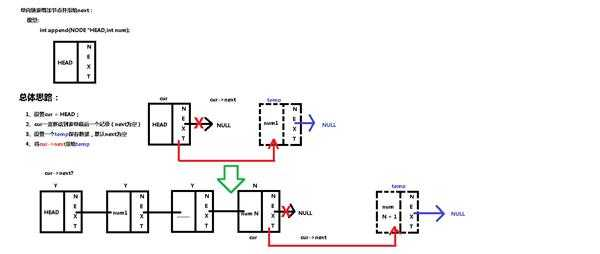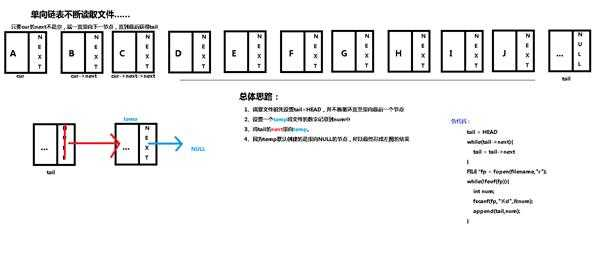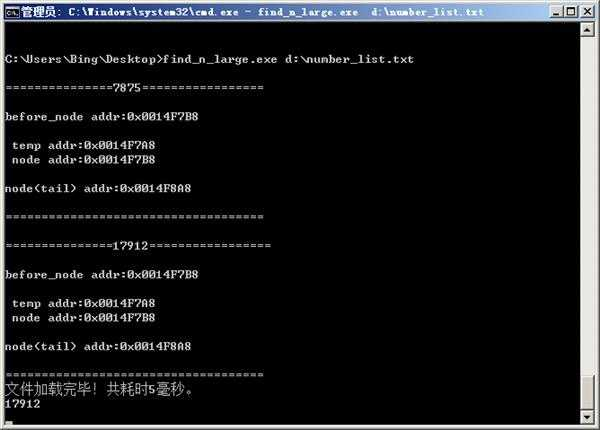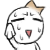﻿ 额……链表读文件的过程中本人DEBUG发现地址总是有偏移（不对） | Code Bye

# 额……链表读文件的过程中本人DEBUG发现地址总是有偏移（不对）

5年前 (2017-04-30) 918次浏览

```typedef struct Node{
int num;
struct Node *next;
}
``````/*追加节点*/
if (!HEAD || num < 0) {
return 0;
}
/*cur一直遍历到最后*/
while (cur->next) {
cur = cur->next;
}
/*设置一个temp保存数据*/
NODE *temp = create_empty();
set_num(temp,num);
/*将cur的next指向temp*/
cur->next = temp;
return 1;
}``````void file_append(NODE *node,int num) {
if (!node) {
return;
}
NODE *temp = create_empty();
temp->num = num;
node->next = temp;
/*这样应该保证每次node都是最后一个节点?*/
node = node->next;
}
int file_input(NODE *HEAD,const char *filename) {
return 0;
}
while (tail->next) {
tail = tail->next;
}
/*这样一来，tail最终指向了最后一个节点*/
FILE *fp = fopen(filename, "r");
if (!fp) {
return 0;
}
while (!feof(fp)) {
int temp;
fscanf(fp, "%d", &temp);
printf("\n===============%d=================\n",temp);
file_append(tail, temp);
printf("\n====================================\n");
}
fclose(fp);
return 1;
}
int main(int argc,char *argv[]) {
if (argc < 2) {
printf("请指明文件名\n");
getchar();
return 1;
}
clock_t start, end;
start = clock();
printf("文件不存在！\n");
getchar();
return 2;
}
end = clock();
printf("文件加载完毕！共耗时%d毫秒。\n",end - start);
getchar();
return 0;
}
```20

http://edu.csdn.net/course/detail/2344 C语言指针与汇编内存地址-一.代码要素

20

```void file_append(NODE **node,int num) {
if (!*node) {
return;
}
NODE *temp = create_empty();
temp->num = num;
(*node)->next = temp;
/*这样应该保证每次node都是最后一个节点?*/
(*node) = temp;
}
//...
file_append(&tail, temp);```

5

Quote: 引用:
```void file_append(NODE **node,int num) {
if (!*node) {
return;
}
NODE *temp = create_empty();
temp->num = num;
(*node)->next = temp;
/*这样应该保证每次node都是最后一个节点?*/
(*node) = temp;
}
//...
file_append(&tail, temp);```5

Quote: 引用:

```NODE *file_append(NODE **node,int num){
/*............*/
return (*node);
}
```

……………………

CodeBye 版权所有丨如未注明 , 均为原创丨本网站采用BY-NC-SA协议进行授权 , 转载请注明额……链表读文件的过程中本人DEBUG发现地址总是有偏移（不对）

[1034331897@qq.com]